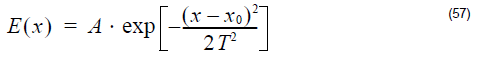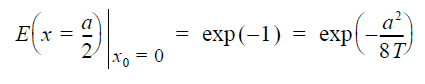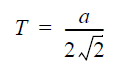In OptiFDTD, transverse Gaussian Beam is expressed aswhere x0 is the center position and T is being called as half width.

In general, Gaussian Beam radius is the radius at which the field amplitude and
intensity drop to 1/e and 1 / e2, respectively. Gaussian Beam Size or Gaussian
Beam Spot size is the beam diameter which is two times of Beam Radius. Suppose
the beam size is denoted as a, which meansThis meansPlease use the above formula to set the half width in OptiFDTD for your desired
Gaussian Beam size.